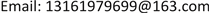﻿ 腐败现象形成机理与奖惩机制研究 Study on the Formation Mechanism of Corruption and the Mechanism of Reward and Punishment

Vol. 07  No. 11 ( 2018 ), Article ID: 27626 , 9 pages
10.12677/AAM.2018.711162

Study on the Formation Mechanism of Corruption and the Mechanism of Reward and Punishment

Xueli Chen, Jicheng Hou

Beijing University of Information Technology, BeijingReceived: Oct. 23rd, 2018; accepted: Nov. 13th, 2018; published: Nov. 20th, 2018ABSTRACT

Corruption has always been the object of attention and research in many countries. Like other countries, China has been doing its part in preventing and punishing corruption. Severe penalties, such as the death penalty, have even been promulgated, but there’s still no effective control of corruption, so we have to rethink what kind of mechanism we can put in place to better reduce or even eliminate corruption. This paper starts from a new way of thinking; a four-party evolutionary game model, including corruption, investigation and punishment, third party intervention mechanism and public opinion supervision, is established by using evolutionary games. By analyzing the game model, this paper provides a valuable reference for the improvement of the mechanism of prevention and punishment of corruption.

Keywords:Corruption, Evolutionary Game, Replication Dynamics1. 引言

2. 腐败现象四方演化博弈模型构建

2.1. 模型假设

2.2. 演化博弈收益矩阵Table 2. The income Matrix of asymmetric Evolutionary Game on Corruption

2.3. 复制动态方程求解

${n}_{t}\left({x}_{i}\right)={N}_{t}\left({x}_{i}\right)/\underset{{x}_{j}\in M}{\sum }{N}_{t}\left({x}_{j}\right)$ (1)

${u}_{t}\left({x}_{i}\right)=\underset{{x}_{j}\in M}{\sum }{n}_{t}\left({x}_{i}\right){u}_{t}\left({x}_{i},{x}_{j}\right)$ (2)

$\stackrel{¯}{{u}_{t}}=\underset{{x}_{i}\in M}{\sum }{n}_{t}\left({x}_{i}\right)\cdot {u}_{t}\left({x}_{i}\right)$ (3)

${{N}^{\prime }}_{t}\left({x}_{i}\right)=k\cdot {N}_{t}\left({x}_{i}\right)\cdot {u}_{t}\left({x}_{i}\right)$ ，( $k>0$ )为常数 (4)

$\text{d}{n}_{t}\left({x}_{i}\right)/\text{d}t={{N}^{\prime }}_{t}\left({x}_{i}\right){\underset{{x}_{j}\in M}{\sum }{N}_{t}\left({x}_{j}\right)-{N}_{t}\left({x}_{i}\right)\underset{{x}_{j}\in M}{\sum }{{N}^{\prime }}_{t}\left({x}_{j}\right)/\left[\underset{{x}_{j}\in M}{\sum }{N}_{t}\left({x}_{j}\right)\right]}^{2}$ (5)

$\text{d}{n}_{t}\left({x}_{i}\right)/\text{d}t=k\cdot {n}_{t}\left({x}_{i}\right)\left[{u}_{t}\left({x}_{i}\right)-\stackrel{¯}{{u}_{t}}\right]$ (6)

2.3.1. 下级官员复制动态分析(7)

$\left\{\begin{array}{l}{u}_{11}={A}_{2}\\ {u}_{12}={A}_{2}+{C}_{3}+\left(1-\alpha \right){C}_{r}\left(yz-y-z\right)-\alpha C\end{array}$ (8)

$\stackrel{¯}{{u}_{1}}=x\cdot {u}_{11}+\left(1-x\right){u}_{12}$ (9)

$\stackrel{¯}{{u}_{1}}={A}_{2}+\left(1-x\right)\left[\left(1-\alpha \right){C}_{r}\left(yz-y-z\right)+{C}_{3}-\alpha {C}_{r}\right]$ (10)

$F=\text{d}x/\text{d}t=kx\left({u}_{11}-\stackrel{¯}{{u}_{1}}\right)=kx\left(1-x\right)\left\{{C}_{r}\left[\alpha -\left(1-\alpha \right)\left(yz-y-z\right)\right]-{C}_{3}\right\}$ (11)

2.3.2. 上级官员复制动态分析

$\left\{\begin{array}{c}{u}_{21}=\left({A}_{1}-{C}_{1}\right)\left\{x\left[z\alpha +\left(1-z\right)\alpha +z\left(1-\alpha \right)+\left(1-z\right)\left(1-\alpha \right)\right]\right\}+\left({A}_{1}-{C}_{1}+{C}_{2}\right)\\ \left\{\left(1-x\right)\left[\alpha +\left(1-z\right)\alpha +z\left(1-\alpha \right)+\left(1-z\right)\left(1-\alpha \right)\right]\right\}\\ {u}_{22}={A}_{1}\left\{x\left[z\alpha +\left(1-z\right)\alpha +z\left(1-\alpha \right)+\left(1-z\right)\left(1-\alpha \right)\right]+\left(1-z\right)\left(1-\alpha \right)\left(1-x\right)\right\}\\ +\left({A}_{1}-G\right)\left\{\left(1-x\right)\left[z\alpha +\left(1-z\right)\alpha +z\left(1-\alpha \right)\right]\right\}\end{array}$ (12)

$\left\{\begin{array}{l}{u}_{21}={A}_{1}-{C}_{1}+{C}_{2}\left(1-x\right)\\ {u}_{22}={A}_{1}-\left(1-x\right)G\left(\alpha +z-z\alpha \right)\end{array}$ (13)

$\stackrel{¯}{{u}_{2}}=y\cdot {u}_{21}+\left(1-y\right)\cdot {u}_{22}$ (14)

$\stackrel{¯}{{u}_{2}}={A}_{1}+\left(1-x\right)\left(y-1\right)G\left(\alpha +z-z\alpha \right)-y\left[{C}_{1}-{C}_{2}\left(1-x\right)\right]$ (15)

$G=\text{d}y/\text{d}t=ky\left({u}_{21}-\stackrel{¯}{{u}_{2}}\right)=ky\left(1-y\right)\left\{\left(1-x\right)\left[G\left(\alpha +z-z\alpha \right)+{C}_{2}\right]-{C}_{1}\right\}$ (16)

2.3.3. 监察机关复制动态分析

$\left\{\begin{array}{c}{u}_{31}=\left({A}_{3}-{C}_{4}\right)\left[xy\alpha +x\left(1-y\right)\alpha +xy\left(1-\alpha \right)+x\left(1-y\right)\left(1-\alpha \right)\right]+\left({A}_{3}+{C}_{3}+{C}_{r}-{C}_{2}-{C}_{4}-Q\right)\\ \left[\left(1-x\right)y\alpha +\left(1-x\right)y\left(1-\alpha \right)\right]+\left[\left(1-x\right)\left(1-y\right)\alpha +\left(1-x\right)\left(1-y\right)\left(1-\alpha \right)\right]\left({A}_{3}+{C}_{3}+{C}_{r}-{C}_{4}-Q\right)\\ {u}_{32}=\left[xy\alpha +x\left(1-y\right)\alpha +xy\left(1-\alpha \right)+x\left(1-y\right)\left(1-\alpha \right)\right]\cdot {A}_{3}+\left({A}_{3}-Q-R\right)\\ \left[\left(1-x\right)y\alpha +\left(1-x\right)y\left(1-\alpha \right)+\left(1-x\right)\left(1-y\right)\alpha \right]+\left(1-x\right)\left(1-y\right)\left(1-\alpha \right)\left({A}_{3}-Q\right)\end{array}$ (17)

$\left\{\begin{array}{l}{u}_{31}=\left(1-x\right)\left({C}_{3}+{C}_{r}-Q-y{C}_{2}\right)+{A}_{3}-{C}_{4}\\ {u}_{32}={A}_{3}-\left(1-x\right)\left[Q+R\left(\alpha +y-y\alpha \right)\right]\end{array}$ (18)

$\stackrel{¯}{{u}_{3}}=z{u}_{31}+\left(1-z\right){u}_{32}$ (19)

$\stackrel{¯}{{u}_{3}}=\left(1-x\right)\left[z\left({C}_{3}+{C}_{r}-y{C}_{2}\right)-\left(1-z\right)R\left(\alpha +y-y\alpha \right)-Q\right]+{A}_{3}-z{C}_{4}$ (20)

$H=\text{d}z/\text{d}t=kz\left({u}_{31}-\stackrel{¯}{{u}_{3}}\right)=kz\left(1-z\right)\left\{\left(1-x\right)\left[{C}_{3}+{C}_{r}+R\left(\alpha -y\alpha \right)+\left(R-{C}_{2}\right)y\right]-{C}_{4}\right\}$ (21)

2.3.4. 舆论监督复制动态方程

$\left\{\begin{array}{l}{u}_{41}=\left(-{C}_{5}+\text{π}-S\right)\left[\left(1-x\right)yz+\left(1-x\right)\left(1-y\right)z+\left(1-x\right)y\left(1-z\right)+\left(1-x\right)\left(1-y\right)\left(1-z\right)\right]\\ {u}_{42}=\left(-S\right)\left[\left(1-x\right)yz+\left(1-x\right)\left(1-y\right)z+\left(1-x\right)y\left(1-z\right)+\left(1-x\right)\left(1-y\right)\left(1-z\right)\right]\end{array}$ (22)

$\left\{\begin{array}{l}{u}_{41}=\left(1-x\right)\left(-{C}_{5}+\text{π}-S\right)\\ {u}_{42}=\left(1-x\right)\left(-S\right)\end{array}$ (23)

$\stackrel{¯}{{u}_{4}}=\alpha {u}_{41}+\left(1-\alpha \right){u}_{42}$ (24)

$\stackrel{¯}{{u}_{4}}=\left(1-x\right)\left[\alpha \left(\text{π}-{C}_{5}\right)-S\right]$ (25)

$K=\text{d}\alpha /\text{d}t=k\alpha \left({u}_{41}-\stackrel{¯}{{u}_{4}}\right)=k\alpha \left(1-\alpha \right)\left(1-x\right)\left(\text{π}-{C}_{5}\right)$ (26)

2.4. 复制动态稳定性分析

2.4.1. 下级官员稳定性分析

2.4.2. 上级官员稳定性分析

$\alpha >{C}_{1}-\left({C}_{2}+zG\right)\left(1-x\right)/G\left(1-x\right)\left(1-z\right)$ 时， ${\text{d}G\left(y\right)/\text{d}y}_{|y=0}>0$${\text{d}G\left(y\right)/\text{d}y}_{|y=1}<0$ ，此时 $y=1$ 为平衡点， $y=0$ 不是平衡点，上级官员的复制动态会趋向于 ${y}^{*}=1$ ，即博弈方都采用“查处”策略； $\alpha <{C}_{1}-\left({C}_{2}+zG\right)\left(1-x\right)/G\left(1-x\right)\left(1-z\right)$${\text{d}G\left(y\right)/\text{d}y}_{|y=0}<0$${\text{d}G\left(y\right)/\text{d}y}_{|y=1}>0$ ，此时 $y=0$ 为平衡点， $y=1$ 不是平衡点，上级官员的复制动态会趋向于 ${y}^{*}=0$ ，即博弈方都采用“不查处”策略。

2.4.3. 监察机关稳定性分析

$\alpha \ne {C}_{4}-\left(1-x\right)\left[\left(R-{C}_{2}\right)y+{C}_{3}+{C}_{r}\right]/\left(1-x\right)\left(1-y\right)R$ ，令 $H\left(z\right)=0$$z=0,z=1$ 为z的两个稳定状态；由于 $\left(1-x\right)\left(1-y\right)R>0$$\text{d}H/\text{d}z=k\left(1-2z\right)\left\{\left(1-x\right)\left[{C}_{3}+{C}_{r}+R\left(\alpha -y\alpha \right)+\left(R-{C}_{2}\right)y\right]-{C}_{4}\right\}$ ，有以下两种情况： $\alpha >{C}_{4}-\left(1-x\right)\left[\left(R-{C}_{2}\right)y+{C}_{3}+{C}_{r}\right]/\left(1-x\right)\left(1-y\right)R$ 时，有 $\text{d}H\left(z\right)/\text{d}{z}_{|z=0}>0,\text{d}H\left(z\right)/\text{d}{z}_{|z=1}<0$ ，此时 $z=1$ 为平衡点， $z=0$ 不是平衡点，监察官员的复制动态会趋向于 ${z}^{*}=1$ ，即博弈方都采用“监察”策略； $\alpha <{C}_{4}-\left(1-x\right)\left[\left(R-{C}_{2}\right)y+{C}_{3}+{C}_{r}\right]/\left(1-x\right)\left(1-y\right)R$ 时，有 $\text{d}H\left(z\right)/\text{d}{z}_{|z=0}<0,\text{d}H\left(z\right)/\text{d}{z}_{|z=1}>0$ ，此时 $z=0$ 为平衡点， $z=1$ 不是平衡点，监察官员的复制动态会趋向于 ${z}^{*}=0$ ，即博弈方都采用“不监察”策略。

2.4.4. 舆论监督稳定性分析

2.5. 四方博弈复制动态均衡解分析

${D}_{1}={C}_{r}\left(1-\alpha \right)\left(yz-y-z\right)+{C}_{3}-\alpha {C}_{r}$

${D}_{2}=\left(1-x\right)\left[G\left(\alpha +z-z\alpha \right)+{C}_{2}\right]-{C}_{1}$

${D}_{3}=\left(1-x\right)\left[{C}_{3}+{C}_{r}+R\left(\alpha -y\alpha \right)+\left(R-{C}_{2}\right)y\right]-{C}_{4}$

${D}_{4}=\left(1-x\right)\left(P-{C}_{5}\right)$

${\theta }_{2}={C}_{1}-\left(1-x\right)\left({C}_{2}+Gz\right)/\left(1-x\right)\left(1-z\right)G$

${\theta }_{3}={C}_{4}-\left(1-x\right)\left[\left(R-{C}_{2}\right)y+{C}_{3}+{C}_{r}\right]/\left(1-x\right)\left(1-y\right)R$

3. 结论

1) 提高上级领导查处腐败现象后所得收益 ${C}_{2}$ ，这里多指经济效益，如此上级领导会更乐于对腐败现象进行查处，理性的下级官员更不会冒着更高的被查处风险选择腐败，这也侧面说明了“高薪养廉”  思想的重要性，关于这方面可借鉴其他国家做法。

2) 加重对下级官员腐败行为的经济处罚 ${C}_{r}$  ，这样腐败者才会因为影响到自身实际利益而有所收敛，所以应完善相关法律法规，寻找摸索最符合自身国情的有效措施。

3) 提高对上级官员不查处腐败的经济惩处 $G$ ，现实中存在不少官官相护、上级官员包庇下级官员腐败甚至伙同参与腐败等一系列不正风气，所以需完善监察政策  ，不单单要惩处下级官员，对待不认真查处腐败现象的上级官员更要给予严厉的惩罚，让官员们感觉到时刻身处监督中而不敢也不能腐败。

Study on the Formation Mechanism of Corruption and the Mechanism of Reward and Punishment[J]. 应用数学进展, 2018, 07(11): 1393-1401. https://doi.org/10.12677/AAM.2018.711162

1. 1. 朱江南, 王燕燕. 严刑能遏制腐败吗?——以中国为例的博弈论分析[J]. 经济社会体制比较, 2013(2): 145-153.

2. 2. 宋余超. 网络舆情形成阶段主体博弈模型研究[D]: [硕士学位论文]. 福州: 福州大学, 2016.

3. 3. 俞建. 博弈论选讲[M]. 北京: 科学出版社, 2014: 47-60.

4. 4. 谢识予. 经济博弈论(第三版) [M]. 上海: 复旦大学出版社, 2012: 210-246.

5. 5. 徐建中, 徐莹莹. 基于演化博弈的制造企业低碳技术采纳决策机制研究[J]. 运筹与管理, 2014(5): 264-272.

6. 6. Dieckmann, U. and Law, R. (1996) The Dynamical Theory of Coevolution: A Derivation from Stochastic Ecological Processes. Journal of Mathematical Biology, 34, 579-612. https://doi.org/10.1007/BF02409751

7. 7. 李芳. 成本与收益理论在预防贿赂犯罪中的应用研究[D]: [硕士学位论文]. 长沙: 湖南大学, 2010: 1-3.

8. 8. 金明军. 我国基层干部腐败防治问题研究[D]: [硕士学位论文]. 大庆: 东北石油大学, 2016: 28-33.

9. 9. 易刚强. 基于演化博弈理论的公职人员腐败及监督方反腐败的行为选择机理研究[D]: [硕士学位论文]. 成都: 西南财经大学, 2014.